# How to use MID function in Microsoft Excel

In this article, we will learn how we use MID function in Microsoft Excel.

MID function returns the specific number of characters from the middle of a string, based on the starting position and the number of characters we specify.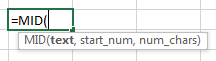Let’s take an example and understand:-

Here, we have some sample data string in column A. And, we will extract the result in column B. Here we have included 7 examples to understand different ways of using MID function.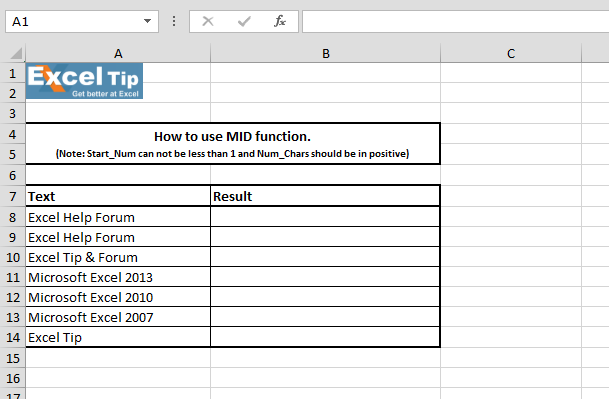1st Example:-

In this example, we will learn how to use MID function and will retrieve 1st text string from the cell.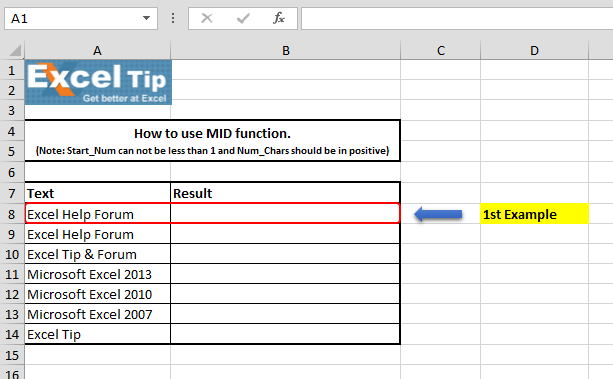• Enter the function in cell B8
• =MID(A8,1,5)
• Press Enter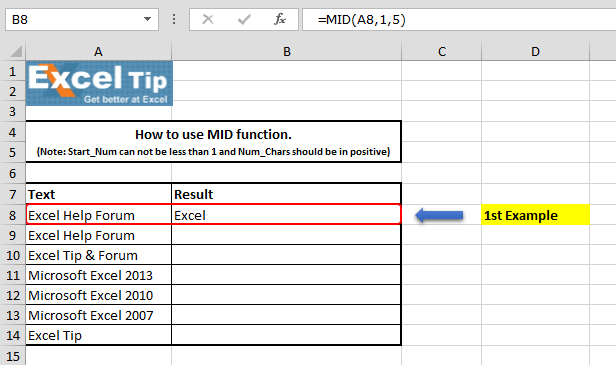Formula Explanation:- This function takes 3 arguments which are text, start_num, and [num_chars]; in this example, as we want to retrieve Excel for the text string that is why we have mentioned 1 for start number and for end number we have defined 5.

2nd Example:-

In this example, we will retrieve 2nd text string from the cell.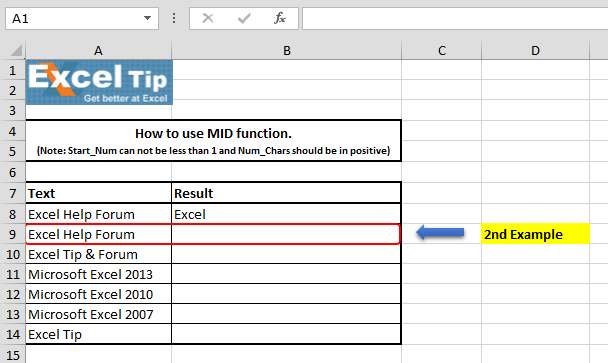• Enter the function in cell B9
• =MID(A9,7,4)
• Press Enter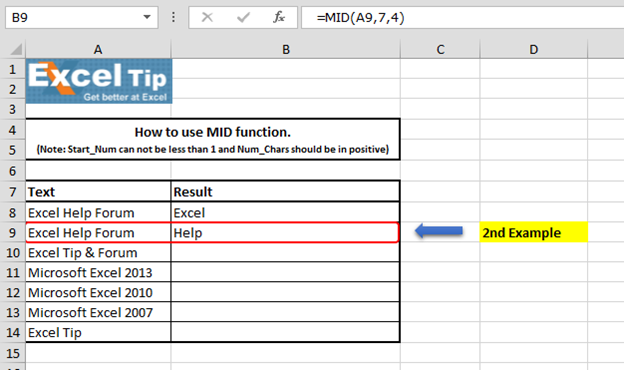Formula Explanation:-   In the above image, we can see that function has returned “Help”. Our second text string is starting from the 7th place and for the second argument our 7th place will be counted as 1 and then we have defined 2nd argument according to the text string.

3rd Example:-

In this example, we have taken special character and we want to retrieve special character as a result.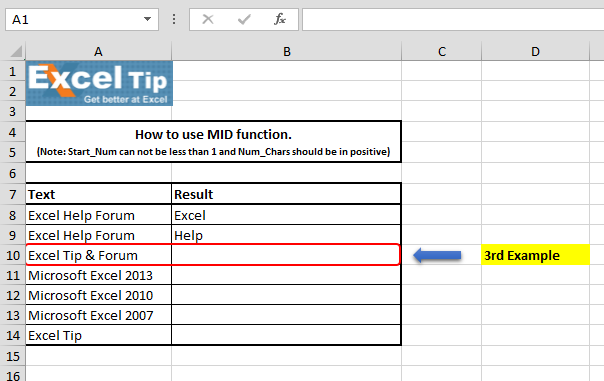• Enter the function in cell B10
• =MID(A10,11,1)
• Press Enter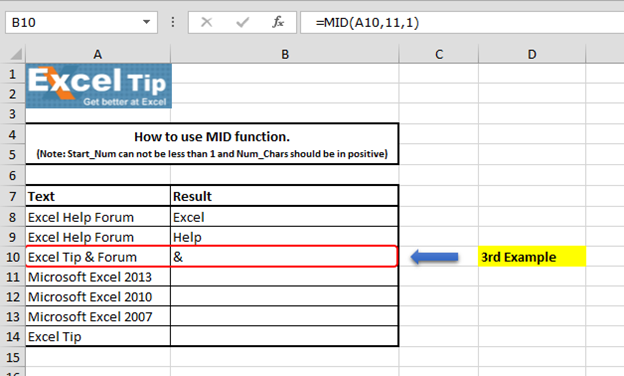Formula Explanation:- In the above image, the special character “&” is at 10th place. So in the example, we have mentioned that our string is starting from 10th place.

4th Example:-

In this example, we’ll see what happens when the start number greater than length of text in the cell is entered.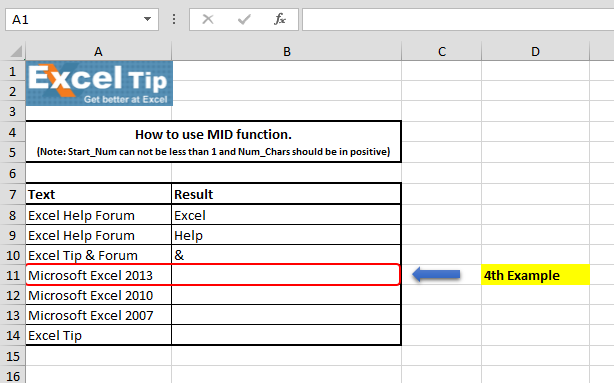• Enter the function in cell B11
• =MID(A11,25,2)
• Press Enter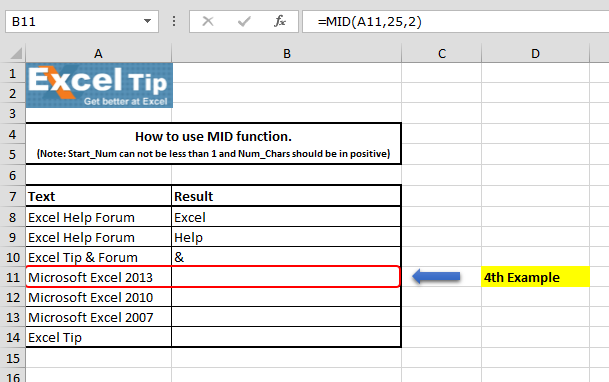The function has returned blank, because it starts from the position of end string.

5th Example:-

In this example, we will enter negative value as starting number to see what happens.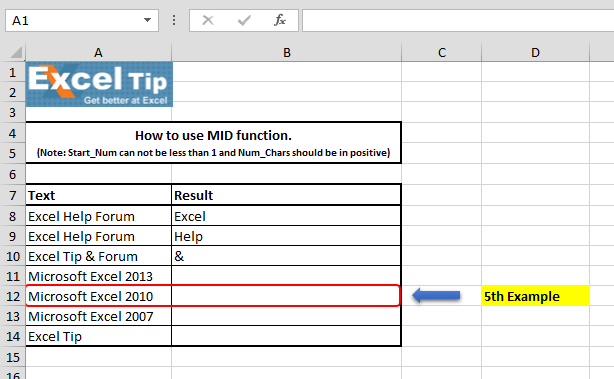• Enter the funcion in cell B12
• =MID(A12,1,-1)
• Press Enter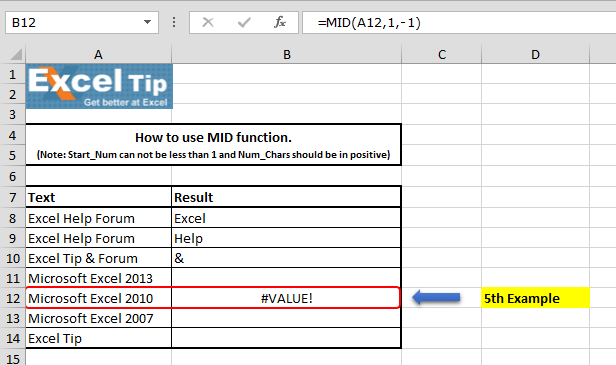• The function will return #VALUE! error, it is because MID function does not allow any negative number or zero as starting number.

6th Example:-

In this example, we will enter zero as number of character, let’s see what happens.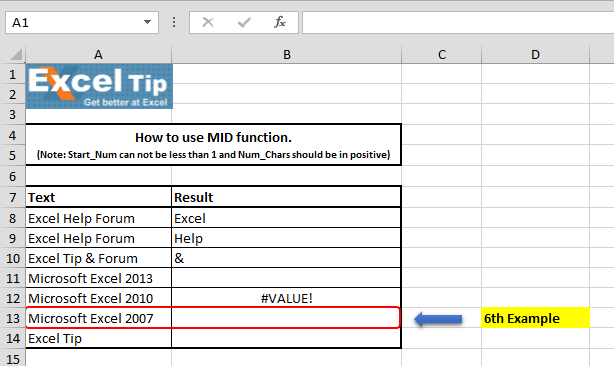• Enter the function in cell B13
• =MID(A13,11,0)
• Press Enter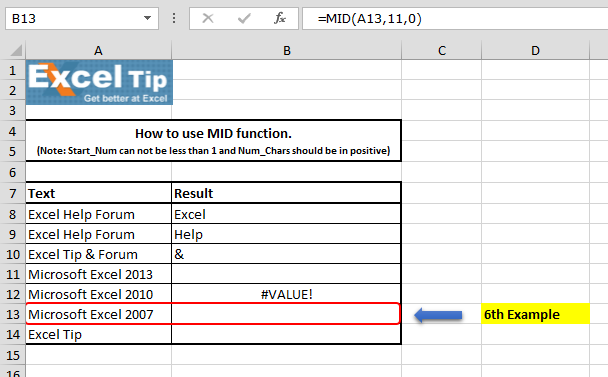The function has returned to an empty cell, because we are telling Excel to start looking from 11th position in cell A13 but do not return any character and thus the function returns empty cell.

7th Example:-

In this example, we will see what happens if we enter number of characters greater than text length.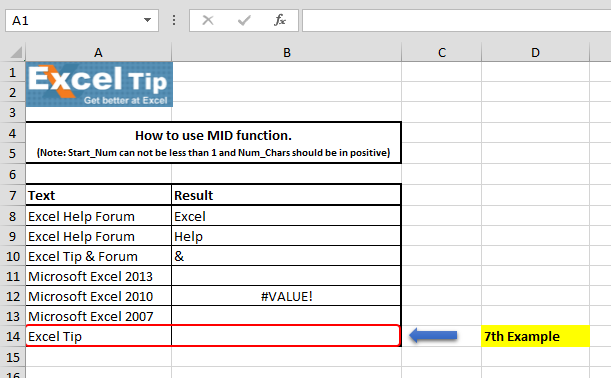• Enter the function in cell B14
• =MID(A14,1,15)
• Press Enter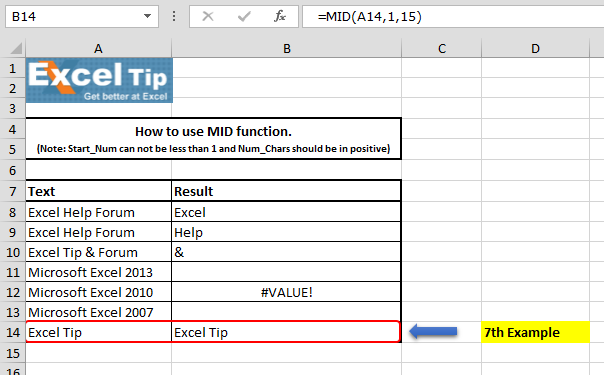We can see in the above image, that function has returned the actual text which is in cell A14 from the starting number.

So, this is how we can use MID function in different ways in Excel.

# Video: How to use MID function in Microsoft Excel

Watch the steps in this short video, and the written instructions are above the video